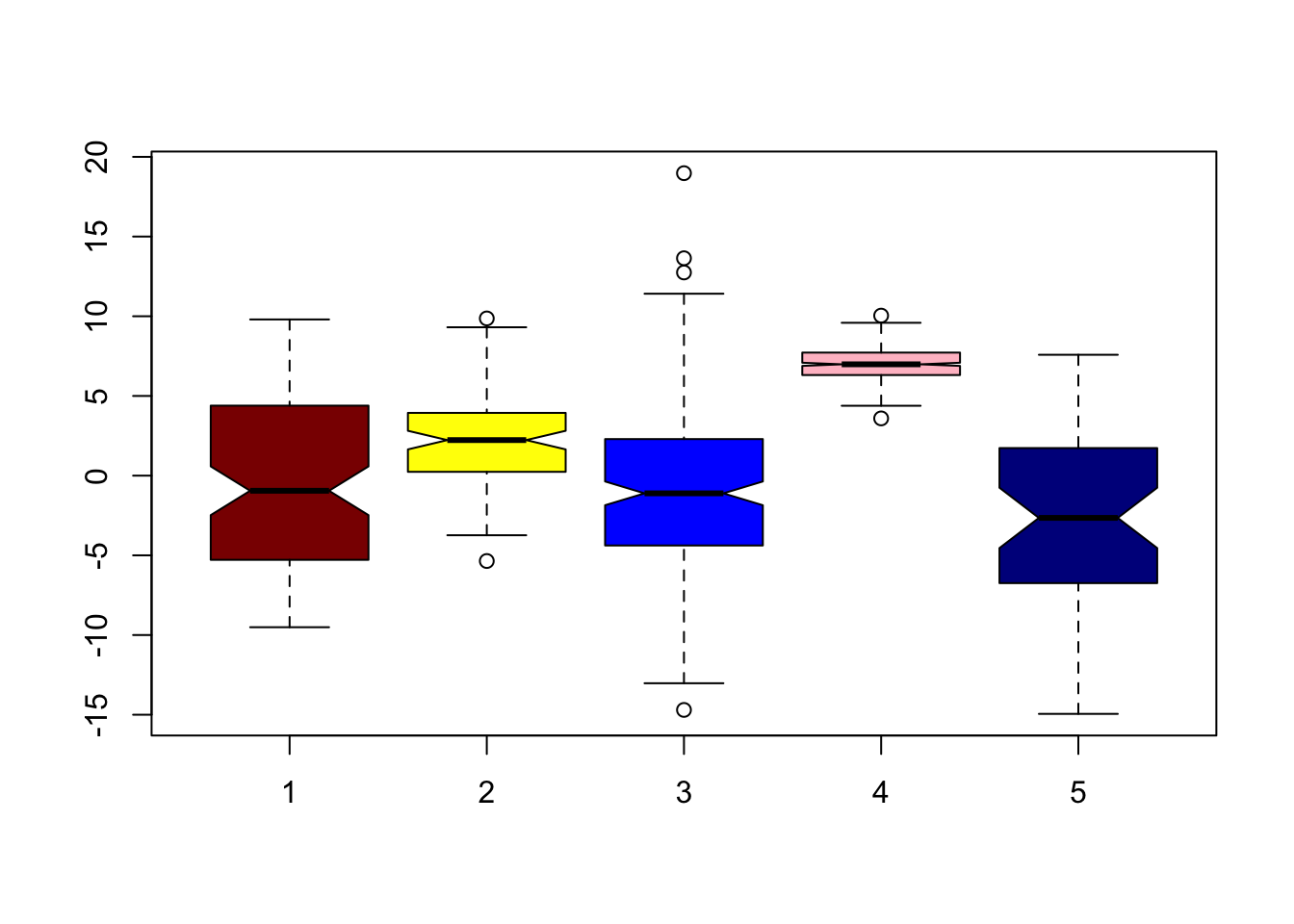Your verification ID is: guDlT7MCuIOFFHSbB3jPFN5QLaQ Big Computing: Boxplot in Base R

## Thursday, October 9, 2014

### Boxplot in Base R

The boxplot is a quick and easy way to visualize the means and quartiles of columns of data or a variables. The plot usually includes plots of any outliers. Although it is rare to be used in such a fashion you can do a boxplot of a single variable.
``````indep<-runif(100,-10,10)
boxplot(indep)``````Alone it is not very useful. A boxplot really shows its use when looking at more than one variable and comparing their means and any quartile overlap. Here I add one normal distrbution with mean 3 and standard deviation of 3 and plot it againt the other variable.
``````nordis<-rnorm(100,3,3)
boxplot(indep,nordis)``````Notice the outliers of the normal distribution that are plotted individually. Lets do one more plot with more variables.
``````n2<-rnorm(200,-1,5)
n3<-rnorm(500,7,1)
n4<-rnorm(50,-2,7)
boxplot(indep,nordis,n2,n3,n4)``````Finally it may be helpful to add some color to the plot. I will also change notch=FALSE to notch=TRUE. As a result a notch is drawn in each of the boxes. If the notches of two plots do not overlap this is ‘strong evidence’ that the two medians differ (Chambers et al, 1983, p. 62).
``boxplot(indep,nordis,n2,n3,n4,notch=TRUE,col=c("dark red","yellow","blue","pink","dark blue"))``